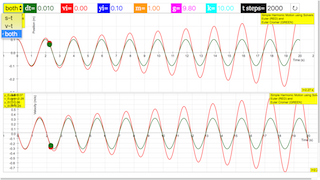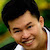Intro Page

Simple Hanging Harmonic Oscillator

Developed by K. Roos

In this set of exercises the student builds a computational model of a hanging mass-spring system that is constrained to move in 1D, using the simple Euler and the Euler-Cromer numerical schemes. The student is guided to discover, by using the model to produce graphs of the position, velocity, and energy of the mass as a function of time, that the Euler algorithm does not conserve energy, and that for this simple oscillatory system, a modified algorithm (Euler-Cromer) is necessary to avoid artificial behavior in the model.

 Subject Area Mechanics First Year and Beyond the First Year C/C++, Fortran, IPython/Jupyter Notebook, Mathematica, Octave*/MATLAB, Python, and Spreadsheet Students who complete this set of exercises will be able to build a model of a simple hanging harmonic oscillator using the Euler algorithm (Exercises 1 and 2); be able to build a model of a simple hanging harmonic oscillator using the Euler-Cromer algorithm (Exercises 4 and 5); be able to produce graphs of the positon, velocity, and total energy as a function of time from the results of their computational model (Exercises 1-5); be able to assess the accuracy of two different computational algorithms (Euler and Euler-Cromer) by comparing results from the different algorithms to each other and to the exact analytical solution (Exercises 1-5); discover that they bloody well can’t use the simple Euler method when modeling an oscillatory system (Exercises 1-3).
Hanging_SHO_Python.py

# Written by:

# Kelly Roos

# Engineering Physics

# This email address is being protected from spambots. You need JavaScript enabled to view it. | 309.677.2997

import numpy as np

import matplotlib.pyplot as plt

import math

# Input parameters for model

dt = 0.01 # time step (s)

vi = 0 # initial velocity (m/s)

yi = 0.1 # initial position (m), relative to eq position yeq (m)

m = 1.0 # mass (kg)

g = 9.8 # gravity (m/s^2)

k = 10 # spring constant (N/m)

t_steps = 2000 # number of time steps

# Defines the 1D arrays to be used in the computation and

# sets all values in the arrays to zero

time = np.zeros(t_steps)

y_Euler = np.zeros(t_steps)

y_EC = np.zeros(t_steps)

y_exact = np.zeros(t_steps)

v_Euler = np.zeros(t_steps)

v_EC = np.zeros(t_steps)

v_exact = np.zeros(t_steps)

energyEuler = np.zeros(t_steps);

energyEC = np.zeros(t_steps);

# Initial conditions

time = 0

y_Euler = yi

y_EC = yi

y_exact = yi

v_Euler = vi

v_EC = vi

v_exact = vi

energyEuler=0.5*m*v_Euler**2+0.5*k*(y_Euler+m*g/k)**2-m*g*(y_Euler+m*g/k)

energyEC=0.5*m*v_EC**2+0.5*k*(y_EC+m*g/k)**2-m*g*(y_EC+m*g/k)

# Main loop: Euler algorithm, euler-Cromer, and evaluation of exact solutions for v and y

for i in range(2, t_steps):

time[i] = time[i-1] + dt

# Euler

v_Euler[i]=v_Euler[i-1]-k*y_Euler[i-1]*dt/m

y_Euler[i]=y_Euler[i-1]+v_Euler[i-1]*dt

# Euler-Cromer

v_EC[i]=v_EC[i-1]-k*y_EC[i-1]*dt/m

y_EC[i]=y_EC[i-1]+v_EC[i]*dt

# Exact (assuming vi=0)

v_exact[i]=-yi*math.sqrt(k/m)*math.sin(math.sqrt(k/m)*time[i])

y_exact[i]=yi*math.cos(math.sqrt(k/m)*time[i])

# Energies

energyEuler[i]=0.5*m*v_Euler[i]**2+0.5*k*(y_Euler[i]+m*g/k)**2-m*g*(y_Euler[i]+m*g/k)

energyEC[i]=0.5*m*v_EC[i]**2+0.5*k*(y_EC[i]+m*g/k)**2-m*g*(y_EC[i]+m*g/k)

# Plotting Results

plt.plot(time, v_Euler, time, v_EC)

plt.ylim((-0.3, 0.3))

plt.xlim((0, 20))

plt.xlabel('time (s)')

plt.ylabel('position (m)')

plt.title('position vs. time')

plt.show()

Exercise 1

Exercise 1: Euler Algorithm Model of a SHO

Build a computational model of a simple hanging harmonic oscillator using the Euler method. Use realistic values for the parameters (i.e., spring constant  and attached mass , such as would be encountered in a typical introductory mechanics laboratory exercise. Also, assume that the mass of the spring is negligible compared to the attached mass, and that the harmonic oscillator has been stretched vertically downward a distance , relative to its hanging equilibrium position and released from rest. Use the model to produce graphs of the position and velocity of the mass as a function of time, and compare these with the exact functions for the position and velocity,

and

that result from solving Newton’s 2nd Law analytically. Does the angular frequency match that expected for a simple harmonic oscillator of mass  an spring constant ?

Exercise 2

Exercise 2: Artificial Behavior with the Euler Algorithm

You may (should!) have noticed that something is not right with the Euler model of your hanging oscillator. Describe in detail the artificial behavior you observe in your model, and explain why it doesn’t represent a realistic oscillating mass. Recall that in the Euler method, the accuracy of the solution can be increased by using a smaller value of . Can you get rid of the artificial behavior by making  smaller?

Exercise 3

Exercise 3: Energy in the Euler Algorithm Model of a SHO

Modify your model to produce a graph of the total energy of the oscillator as a function of time. Describe in detail what happens to the energy, and the artificial behavior observed. Can this artificial behavior in the energy be corrected by making  smaller? What can you conclude about using the Euler method to model a simple harmonic oscillator?

Exercise 4

Exercise 4: Euler-Cromer Algorithm Model of a SHO

Build a model of the hanging oscillator using the modified Euler, or Euler-Cromer, numerical method. Compare the results you obtain (i.e. position and velocity vs. time) with those obtained from the simple Euler method, and with the exact solution. Comment in detail on your results.

Exercise 5

Exercise 5: Energy in the Euler-Cromer Algorithm Model of a SHO

Modify your model to produce a graph of the total energy as a function of time. Is energy conserved for the Euler-Cromer algorithm?

Translations

Code Language Translator RunSoftware Requirements

SoftwareRequirements

 Android iOS Windows MacOS with best with Chrome Chrome Chrome Chrome support full-screen? Yes. Chrome/Opera No. Firefox/ Samsung Internet Not yet Yes Yes cannot work on some mobile browser that don't understand JavaScript such as..... cannot work on Internet Explorer 9 and below

CreditsFremont Teng; Loo Kang Wee

[text]

Hanging Simple Harmonic Oscillator JavaScript Simulation Applet HTML5

Instructions

Graph Combo Box

Toggling between the combo box will give you the respective views.

(s-t Graph)

(v-t Graph)

(Both)

Control Panel

Setting the variables in the control panel will adjust the graphs accordingly.

Reset Button

Resets the Simulation.

Research

[text]

[text]

[text]